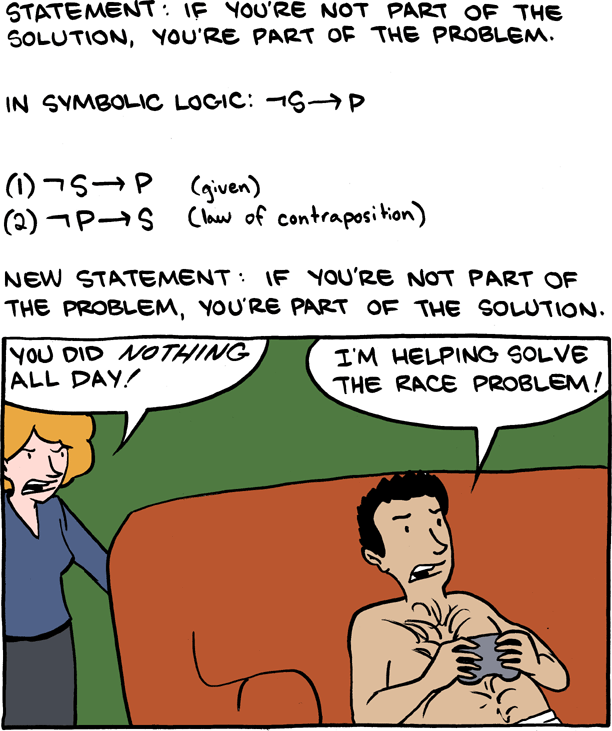When one starts studying logic one is likely to be surprised by the workings of the so-called material implication, p –> q (if p, then q). Unlike the implication used in natural language, which can for example indicate causation, the material implication has a more restricted meaning. The material implication is true unless p is true and q is false. This is ultimately a matter of definition to resolve ambiguities present in natural language. A very simple and short sentence such as “visiting relatives can be boring” can already have two very different meanings. Either it is boring to visit relatives, or relatives that are visiting can be boring. Logic seeks to resolve such ambiguities by explicitly agreeing on a formal interpretation of symbols.

The meaning of the material implication is reflected in this truth table:

p q p -> q
0 0 1
1 0 0
0 1 1
1 1 1

A consequence of this stricter definition of the implication in logic means that sometimes its formal interpretation is at odds with the way implications are interpreted in natural language. This can lead to the experience of a paradox. The part of the material implication that is counter-intuitive to most people is that p -> q is true when p is false, irregardless of whether q is true. This is called a vacuous truth in logic: if p is false, then p -> q is asserting a true property of something that does not occur.

1. ~p -> (p -> q)

Since the material implication is defined as “it is not the case that p is true and q not” ( ~(p /\ ~q) ), the only way we can show that the implication does not hold is by a counterargument where p holds and q not. However, if p never holds, we are not able to give such an argument and it is said that the implication holds vacuously. The implication then is an “empty truth” that is true because we cannot show it to be false, but that does not convey any information.

Consider an analogue example. If you would say to your father that he is your favourite (biological) father, this would be true. But it is equally true that he is your least favourite father, and these two statements thus do not convey any information about the father (or your attitude towards him). Both statements can be thought of as empty truths: the statements are a comparison with another non-existing father and thus are true “automatically”.

We can show some more statements that are counter-intuitive but hold according to this definition of the material implication (see here ). We can see in the above truth table that the material implication is true if p is false. If the expression p is a contradiction, it will always be false. Hence, if we have a contradiction, we can conclude any formula q:

1. (~p /\ p) -> q

Although this is “logical”, this leads to very weird results when translated to natural language. For example: if it rains and it does not rain, then my cat can fly. This is called ex falso sequitur quod libet . Anything follows from a contradiction.

At the same time, we can also see in the truth table that when q is true, the implication always holds, irregardless of the truth of p. But this also sounds a bit counter-intuitive: if q is true, then any p implies q.

1. q -> (p -> q).

For example, when we say in natural language “if I am sick, then I go to the doctor”, we assume there is a clear (causal) relation between these propositions. The above formula then would say: if I go to the doctor, then it holds that if I’m sick, I go to the doctor. That is clear enough. But logically speaking, it is equally true that “if I go to the doctor, then if I’m not sick, I go to the doctor.” This has a quite different ring to it: people usually do not go to the doctor because they are not sick (unless they are hypochondric).

So far we have that p -> q is always true if p is false, or if q is true. Thus we can rewrite p -> q as ~p / q. This follows pretty directly from the definition of the logical implication, i.e. “it is not the case that p is true and q is not true”:

1. ~(p /\ ~q)
2. ~p / ~~q (DeMorgan’s law)
3. ~p / q

You can easily see the equivalence of these formula’s as they have the same truth table:

p q ~p p -> q ~p / q
0 0 1 1 1
1 0 0 0 0
0 1 1 1 1
1 1 0 1 1

We can use this for example to show that if p does not imply q, then p holds and q does not hold.

1. ~(p -> q) -> (p /\ ~q).

This is also quite surprising! For example, this could mean: If Edwin eating a lot of cheese does not imply that Edwin lives in the Netherlands, then Edwin eats a lot of cheese but Edwin does not live in the Netherlands.

We can show that the left and right statement are equivalent, for example as such:

Proof:

• ~(p -> q)
• ~(~p / q) (DeMorgan’s Law)
• (~~p /\ ~q) (Using ~~p entails p)
• p /\ ~q

N.B. ~~p ==> p does not hold in intuistionistic logic.

If you master all of this, you can make (and explain) jokes like these: